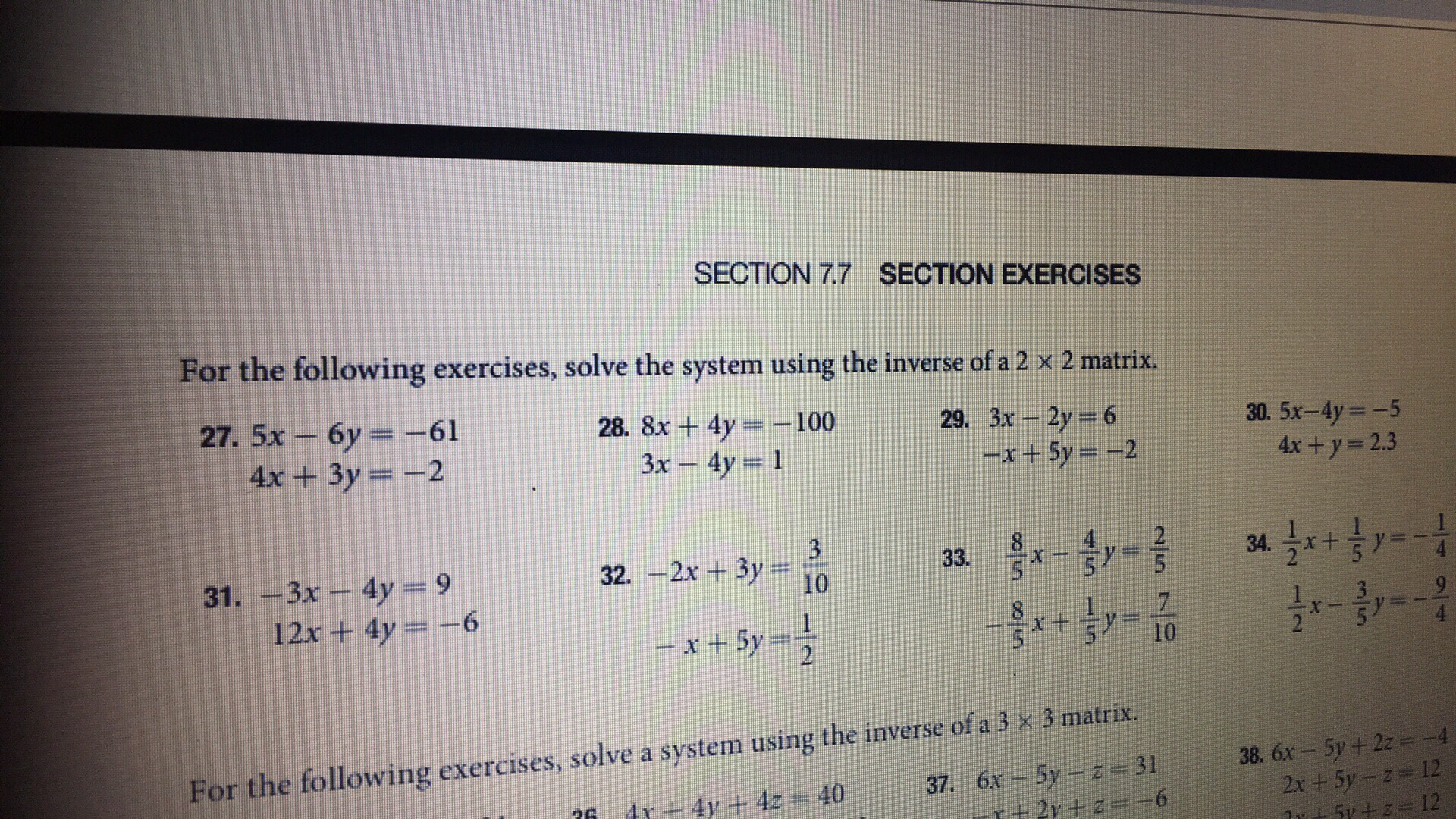# SECTION 7.7SECTION EXERCISESFor the following exercises, solve the system using the inverse of a 2 x 2 matrix.27. 5x-6y =-614x+3y 228. 8x + 4y-:-1003x -4y 129. 3x-2y = 630. 5x-4y -54x+y- 2.3-x + 5y =-231. -3x - 4y 92 512x + 4y =-6For the following exercises, solve a system using the inverse of a 3 x 3 matrix38. 6x- 5y+2z-412

Question
49 views

I need help with #27,29help_outlineImage TranscriptioncloseSECTION 7.7 SECTION EXERCISES For the following exercises, solve the system using the inverse of a 2 x 2 matrix. 27. 5x-6y =-61 4x+3y 2 28. 8x + 4y-:-100 3x -4y 1 29. 3x-2y = 6 30. 5x-4y -5 4x+y- 2.3 -x + 5y =-2 31. -3x - 4y 9 2 5 12x + 4y =-6 For the following exercises, solve a system using the inverse of a 3 x 3 matrix 38. 6x- 5y+2z-4 12 fullscreen
check_circle

Step 1
Step 2
Step 3

### Want to see the full answer?

See Solution

#### Want to see this answer and more?

Solutions are written by subject experts who are available 24/7. Questions are typically answered within 1 hour.*

See Solution
*Response times may vary by subject and question.
Tagged in

### Determinants